# 【图像分割】“最大流-最小割”算法

## 计算机视觉，图像分割，最大流-最小割

Posted by x-jeff on October 26, 2018

# 1.最大流问题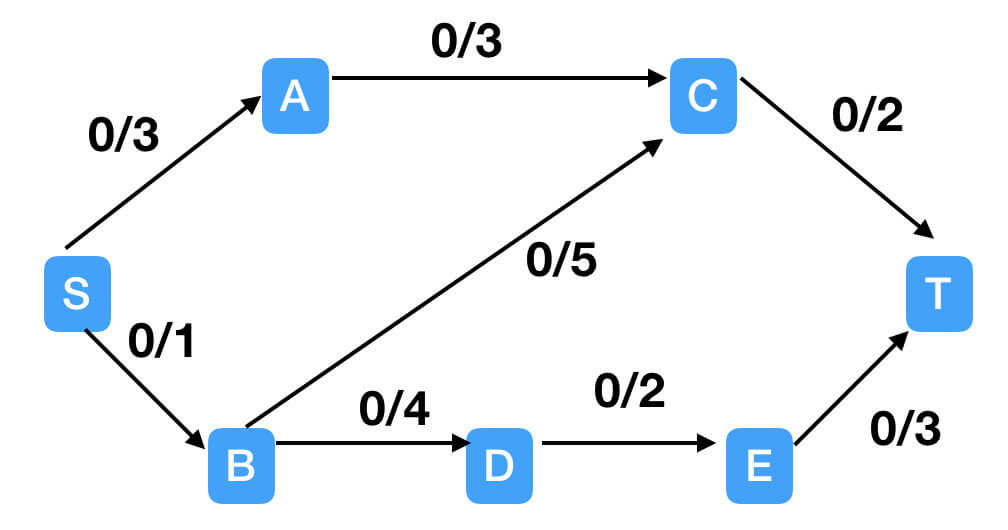如上图所示，如果起点为S点，终点为T点，有水流从S点流向到T点。图中黑色带箭头的直线为管道，水流只能按照箭头的方向前进，很明显，一个管道中不可能有两种方向的水流。“/”前的数字表示该条管道目前的流量，“/”后面的数字表示该条管道能承载的最大流量。水流从S点出发，集结到T点，S点出发的流和进入T点的流应该是相等的。最大流问题就是使流尽可能的大（在满足每条边容量的限制下）。

• 增广路径算法（augmenting paths）
• 预流推进算法（push-relabel）

# 2.增广路径算法

## 2.1.基本术语

$c(u,v)-f(u,v)$为剩余的容量，也称为残量。任意时刻，图中残量不为0的边组成的网络被称为残余网络，该网络上从s到t的路径被称为增广路径

## 2.2.增广路径算法的主体思想

STEP1：将容量网络转化成残余网络。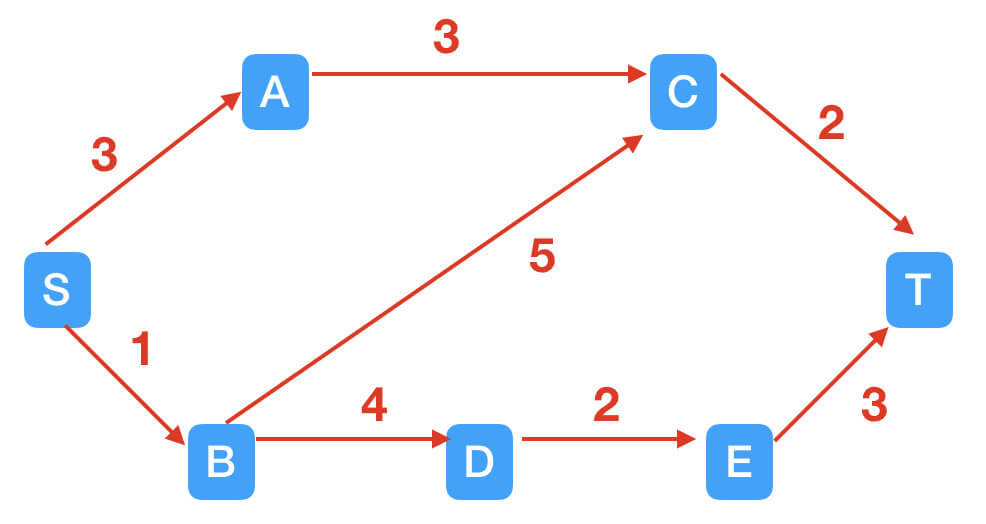STEP2：找到增广路径S$\rightarrow$A$\rightarrow$C$\rightarrow$T。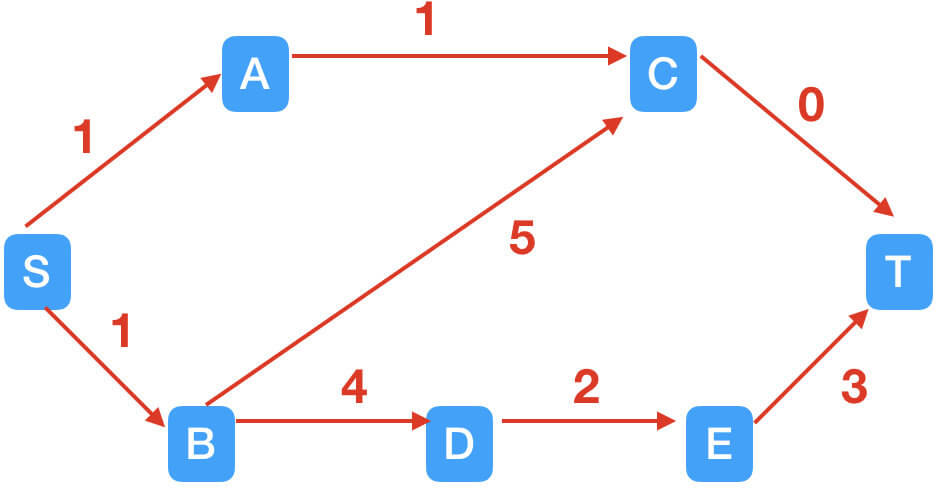STEP3：找到增广路径S$\rightarrow$B$\rightarrow$D$\rightarrow$E$\rightarrow$T。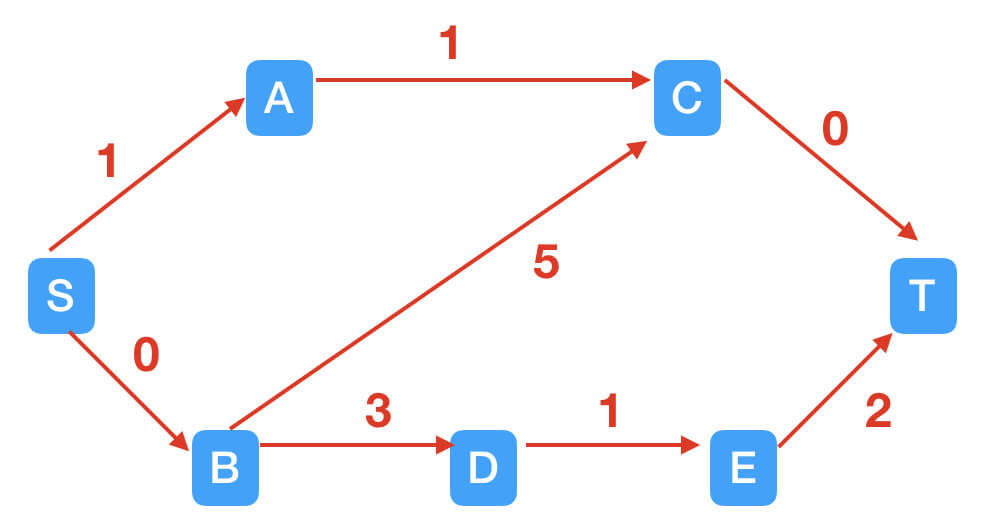## 2.3.Fold-Fulkerson算法

Fold-Fulkerson算法的核心思想就是引入反向边，正向边流了k个单位的流量，其反向边的残量增加k。

STEP1：引入反向边。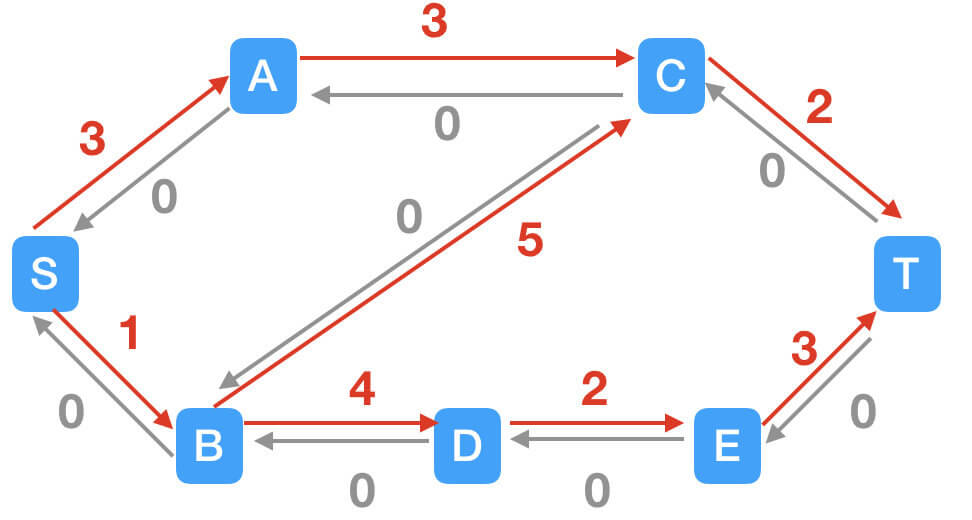STEP2：找到路径S$\rightarrow$B$\rightarrow$C$\rightarrow$T。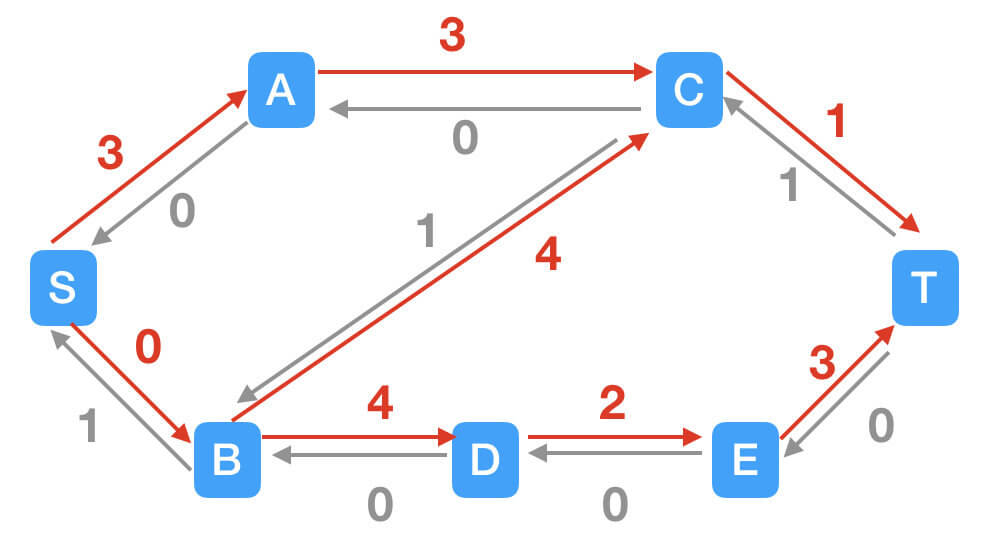STEP3：找到路径S$\rightarrow$A$\rightarrow$C$\rightarrow$T。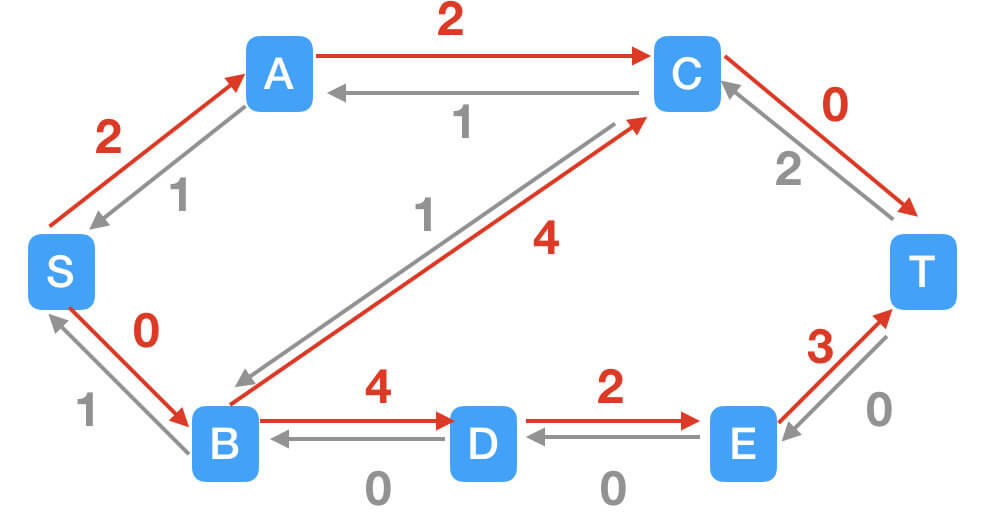STEP4：正向边此时已经不存在增广路径，但是在引入反向边之后，出现了一条新的增广路径：S$\rightarrow$A$\rightarrow$C$\rightarrow$B$\rightarrow$D$\rightarrow$E$\rightarrow$T。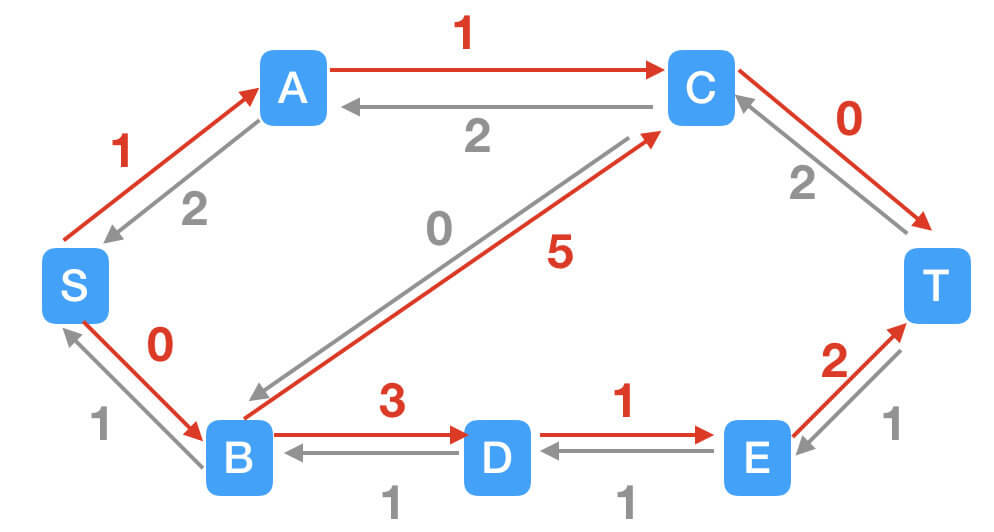## 2.4.搜索路径的方法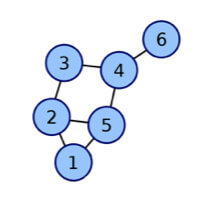• BFS（宽度优先搜索，广度优先搜索）：如果从1开始进行搜索的话，BFS的步骤就是，先搜索所有和1相连的，也就是2和5被找到了，然后再从2开始搜索和他相连的，也就是3被找到了，然后从5搜，也就是4被找到了，然后从3开始搜索，4被找到了，但是4之前已经被5找到了，所以忽略掉就行。然后3开始搜索，忽略4所以啥都没搜到，然后从4开始，6被找到了。
• DFS（深度优先搜索）：DFS的话从1开始，先找到其中一个相连的，2被找到了，然后直接开始从2开始搜索，3被找到了，然后从3开始搜索，4被找到了，然后从4开始搜索，5被找到了，然后从5开始搜索，忽略已经找到的所以啥都没找到。然后没路可走了，回到前面去再走另一条路，从4开始，6被找到了，然后又没路可走了，然后再回去前面4，然后没路了 ，回去前面3，然后一直这样。

# 4.Graph Cut中所用的最大流/最小割算法

OpenCV源码地址👉maxflow

Graph Cut中所用的最大流/最小割算法是在Fold-Fulkerson算法基础上的改进，使用BFS方法寻找路径。接下来让我们一探究竟吧。

## 4.2.新的最小割/最大流算法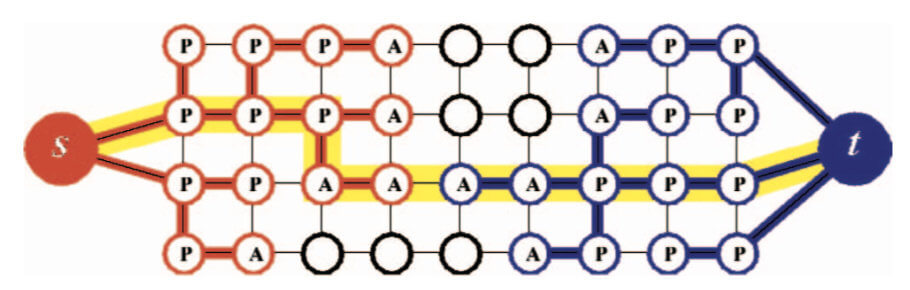如上图所示，构建了两个不重叠的搜索树S和T，有$S\in V,s\in S,T\in V,t\in T,S\cap T=\emptyset$。两棵树的根节点分别是s和t。在树S中，从每个父节点到其子节点的所有边都是不饱和的，而在树T中，从子节点到其父节点的边是不饱和的。（树S中的所有边都是由父节点指向子节点（存在流量），树T中则由子节点指向父节点）。

• “生长”阶段：搜索树S和T扩展节点，直到两树相遇，得到一条由源点s到汇点t的增广路径。
• “增广”阶段：根据找到的增广路径将搜索树拆分为子树或森林。
• “领养”阶段：搜索树S和T重新构建。

### 4.2.1.“生长”阶段(Growth Stage)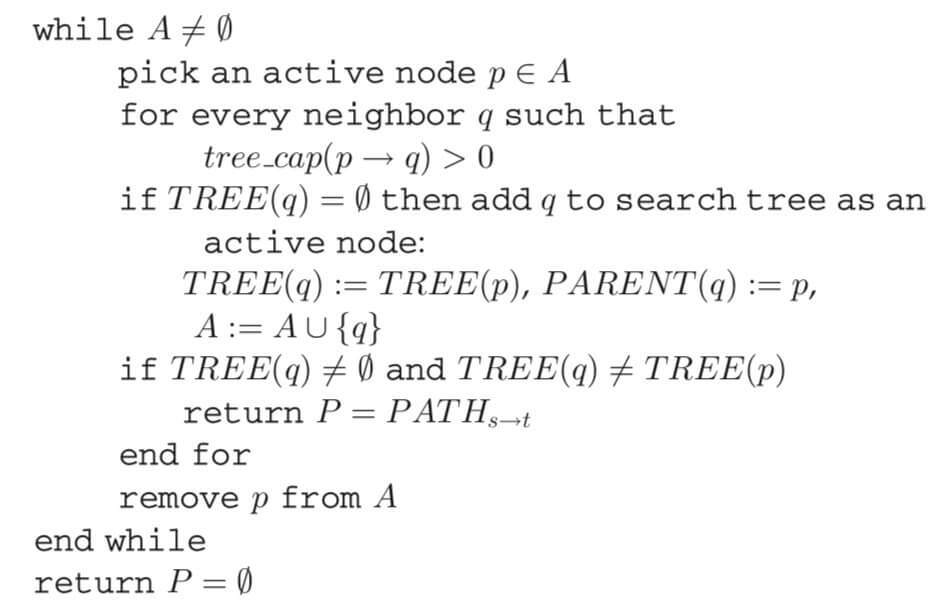while循环执行的条件：$A\neq \emptyset$，即主动节点的集合A不能为空集。

while循环第一步：选择主动节点$p\in A$。

while循环第二步：$q$对$p$的邻居节点，判断tree_cap$(p\rightarrow q)$是否大于0，即是否是饱和边。如果大于0，执行for循环，否则，执行while循环第三步。

for循环第一步：如果$TREE(q)=\emptyset$，则将$q$设为对应搜索树的主动节点，有$TREE(q)=TREE(p),PARENT(q)=p$，将$q$节点加到集合A中，$A=A\cup \lbrace q \rbrace$（符号“:=”的意思是“定义为”）。

for循环第二步：如果$TREE(q)\neq \emptyset$，且$TREE(q)\neq TREE(p)$，说明找到了增广路径，返回$P=PATH_{s\rightarrow t}$。

while循环第三步：将$p$从集合A中移除（经历过for循环之后，节点$p$从主动节点变为被动节点）。

### 4.2.2.“增广”阶段(Augmentation Stage)

“增广”阶段的输入就是“生长”阶段产生的增广路径$P$。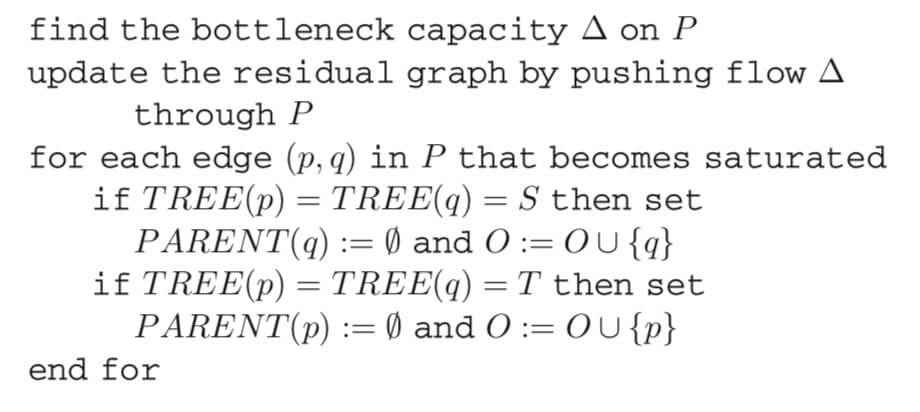第一步：确定路径$P$中所有边的最小残余容量$\Delta$。

for循环判断语句：如果$P$中有边(p,q)变为饱和边，则执行for循环，否则，跳出for循环。

for循环第一步：如果$TREE(q)=TREE(p)=S$，则q没有父节点，即$PARENT(q)=\emptyset$，将q加入到孤儿节点的集合$O$中，即$O=O\cup \lbrace q \rbrace$。

for循环第二步：如果$TREE(q)=TREE(p)=T$，则p没有父节点，即$PARENT(p)=\emptyset$，将p加入到孤儿节点的集合$O$中，即$O=O\cup \lbrace p \rbrace$。

• 属于同一颗树$TREE(q)=TREE(p)$。
• $(q,p)$为不饱和边，即tree_cap$(q\rightarrow p)>0$。
• $q$的“起源“应该是源点s或者汇点t(注意：“起源”不能是其他自由节点或者孤儿节点)。

• 如果tree_cap$(q \rightarrow p)>0$，把$q$ 加到集合A中。
• 如果$PARENT(q)=p$，把$q$加到集合$O$中，同时设置$PARENT(q)=\emptyset$。即自由节点$p$的所有子节点全部变为孤儿节点。

2.$TREE（p）=\emptyset,A=A-\lbrace p \rbrace$。

# 5.参考资料